# GeetCode Hub

We are given the `root` node of a maximum tree: a tree where every node has a value greater than any other value in its subtree.

Just as in the previous problem, the given tree was constructed from an list `A` (`root = Construct(A)`) recursively with the following `Construct(A)` routine:

• If `A` is empty, return `null`.
• Otherwise, let `A[i]` be the largest element of `A`.  Create a `root` node with value `A[i]`.
• The left child of `root` will be `Construct([A, A, ..., A[i-1]])`
• The right child of `root` will be `Construct([A[i+1], A[i+2], ..., A[A.length - 1]])`
• Return `root`.

Note that we were not given A directly, only a root node `root = Construct(A)`.

Suppose `B` is a copy of `A` with the value `val` appended to it.  It is guaranteed that `B` has unique values.

Return `Construct(B)`.

Example 1: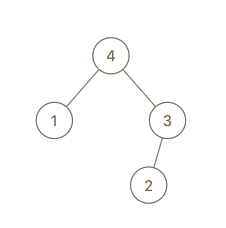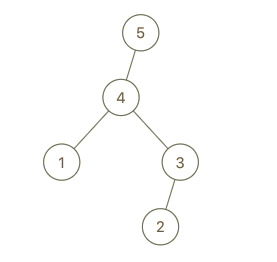```Input: root = [4,1,3,null,null,2], val = 5
Output: [5,4,null,1,3,null,null,2]
Explanation: A = [1,4,2,3], B = [1,4,2,3,5]
```

Example 2: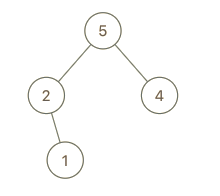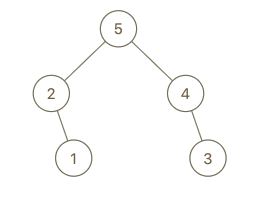```Input: root = [5,2,4,null,1], val = 3
Output: [5,2,4,null,1,null,3]
Explanation: A = [2,1,5,4], B = [2,1,5,4,3]
```

Example 3: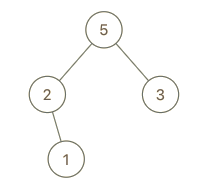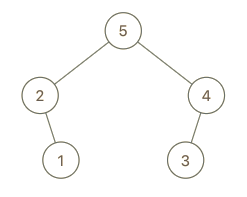```Input: root = [5,2,3,null,1], val = 4
Output: [5,2,4,null,1,3]
Explanation: A = [2,1,5,3], B = [2,1,5,3,4]
```

Constraints:

• `1 <= B.length <= 100`

/** * Definition for a binary tree node. * public class TreeNode { * int val; * TreeNode left; * TreeNode right; * TreeNode() {} * TreeNode(int val) { this.val = val; } * TreeNode(int val, TreeNode left, TreeNode right) { * this.val = val; * this.left = left; * this.right = right; * } * } */ class Solution { public TreeNode insertIntoMaxTree(TreeNode root, int val) { } }